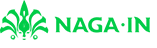@devilogic 2017-07-30T17:17:25.000000Z 字数 4545 阅读 3282

# 玩命的销售日志 2017.7.31

devilogic 日志

#### 谈谈当前移动加固产品的强度

$13$年开始，加固市场慢慢兴起。到现在市场逐步接受这个概念，已经有$4$年时间了。$Android$方面主要有两种文件需要保护。一种是dex，一种是so文件。dex保护方面主要经历了三个阶段。

1. 类加载
2. 类抽取
3. 虚拟机保护

## 谈谈加密算法

#!/usr/bin/python# coding:utf-8import pdbimport numpy as npimport struct#from collections import Counterdef xor(src, key):    f = open('./a.bin', 'wb')    for i in range(0, len(src)):        if src[i] != 0:            m = src[i] ^ key        else:            m = src[i]        f.write(struct.pack('B', m))    f.close()def cracker(src, dest):    """    src 是对比明文    dest 是要破解的密文    """    def __loop_try__(t, d):        r = 0        for k in range(0, 256):            m = d ^ k            if m == t:                r = k                break        return r    data1 = np.fromfile(src, dtype=np.uint8)    data2 = np.fromfile(dest, dtype=np.uint8)    key = 0    keys = {}    for i in range(0, 1000):        key = __loop_try__(data1[i], data2[i])        item = []        if hex(data1[i]) in keys:            item = keys[hex(data1[i])]        if hex(key) not in item:            item.append(hex(key))        keys[hex(data1[i])] = item    return keysif __name__ == "__main__":    keys = cracker('./classes4.dex', './classes.dex')    #data1 = np.fromfile('./classes.dex', dtype=np.uint8)    #xor(data1, 255)    #pdb.set_trace()    print keyselse:    pass

cracker的第一个参数是明文文件，后边是密文文件。

## 总结• 私有
• 公开
• 删除